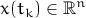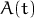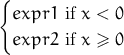### 1 Introduction

#### 1.1 What it does

Runge is an Interactive Solver for Systems of Ordinary Differential Equations. It solves initial value problem (aka Cauchy problem) defined as the following: for a given system of ordinary differential equationsand given initial valuesfind solutionat a given point of “time” i.e. for a given valueof independent variable. Actually Runge produces solutions setwhereis the number of steps taken. This allows to build trajectories of solutions.

#### 1.2 Why Runge?

• It’s fast. It utilizes BLAS and LAPACK FORTRAN libraries optimized for modern multi-core processors.
• It’s interactive. It allows you to start a solution by mouse click on a plane.
• It’s precise. It uses Runge Rule to adjust step length to satisfy required precision on each step.
• It’s effective. When it needs to compute derivatives (Jacobian matrix, for example) it does that analytically, i.e. without using numerical methods.
• It’s portable. It works on Windows and Linux (32 and 64 bit versions) and Mac OSX (64 bit only).
• It’s open. It allows you to implement and embed your own algorithms (aka “solvers”).
• It’s easy to use. It allows to export results to MS Excel and MATLAB.

#### 1.3 System Types and Solvers

Runge comes with pre-installed solvers optimized for solving differential equations of different types:

• Type 1. Generic non-autonomous system• Type 2. Generic autonomous system (it’s a subset of Type 1, i.e. you can use both types 1 and 2 for autonomous sytems — sometimes it makes sense for choosing appropriate solver)• Type 3. Pseudo-linear system (here it’s assumed thatis relatively small compared to matrix)• Type 4. Pseudo-linear system with constant matrix BThe solvers (algorithms) coming in standard package are:

• Runge-Kutta process modification developed by R. England. Fast and precise method of fifth order suitable for solving systems of Type 1. See .
• Exponential method modification developed by J.D. Lawson. It’s recommended for linear and quasi-linear systems of Type 1,3 and 4 (including stiff ones). The method is A-stable for linear systems, i.e. residuals do not depend on step length. See .
• Implicit process developed by H.H. Rosenbrock. It’s recommended for non-linear systems of Type 1 and 2 (including stiff ones). See .

#### 1.4 Expressions and Functions

The following functions and operators are supported for programming the systems mentioned above.

 + - * / ^ arithmetic operators: add, subtract, multiply, divide, power exp(x)sqrt(x)log(x) natural logarithm of x log10(x) common (base 10) logarithm of x sin(x) sine of x cos(x) cosine of x tan(x) tangent of x asin(x) arc sine of x acos(x) arc cosine of x atan(x) arc tangent of x sinh(x) hyperbolic sine of x cosh(x) hyperbolic cosine of x tanh(x) hyperbolic tangent of x sinint(x) sine integral of xcosint(x) cosine integral of xsign(x) sign of xabs(x)iif(x,expr1,expr2) immediate ifsat(x,y) satellite function of x and yi 1 (one) 0 (empty field means zero)

Examples: 2*sin(t-1)+cos(t)-x^2, sqrt(abs(x)), iif(t,sin(x),cos(x)) etc.